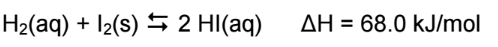# Problem: Considering the following equilibrium, what is the expression for K?a) [HI]2/[H2]b) [H2][I2]/[HI]c) ([H2][I2])1/2 /[HI]2d) [HI]/ [H2]1/2e) [HI]2/[H2][I2]

🤓 Based on our data, we think this question is relevant for Professor Hanlan's class at SFU.

###### Problem Details

Considering the following equilibrium, what is the expression for K?

a) [HI]2/[H2]
b) [H2][I2]/[HI]
c) ([H2][I2])1/2 /[HI]2
d) [HI]/ [H2]1/2
e) [HI]2/[H2][I2]What scientific concept do you need to know in order to solve this problem?

Our tutors have indicated that to solve this problem you will need to apply the Equilibrium Expressions concept. If you need more Equilibrium Expressions practice, you can also practice Equilibrium Expressions practice problems.

What is the difficulty of this problem?

Our tutors rated the difficulty ofConsidering the following equilibrium, what is the expressio...as medium difficulty.

How long does this problem take to solve?

Our expert Chemistry tutor, Sabrina took 2 minutes and 7 seconds to solve this problem. You can follow their steps in the video explanation above.

What professor is this problem relevant for?

Based on our data, we think this problem is relevant for Professor Hanlan's class at SFU.